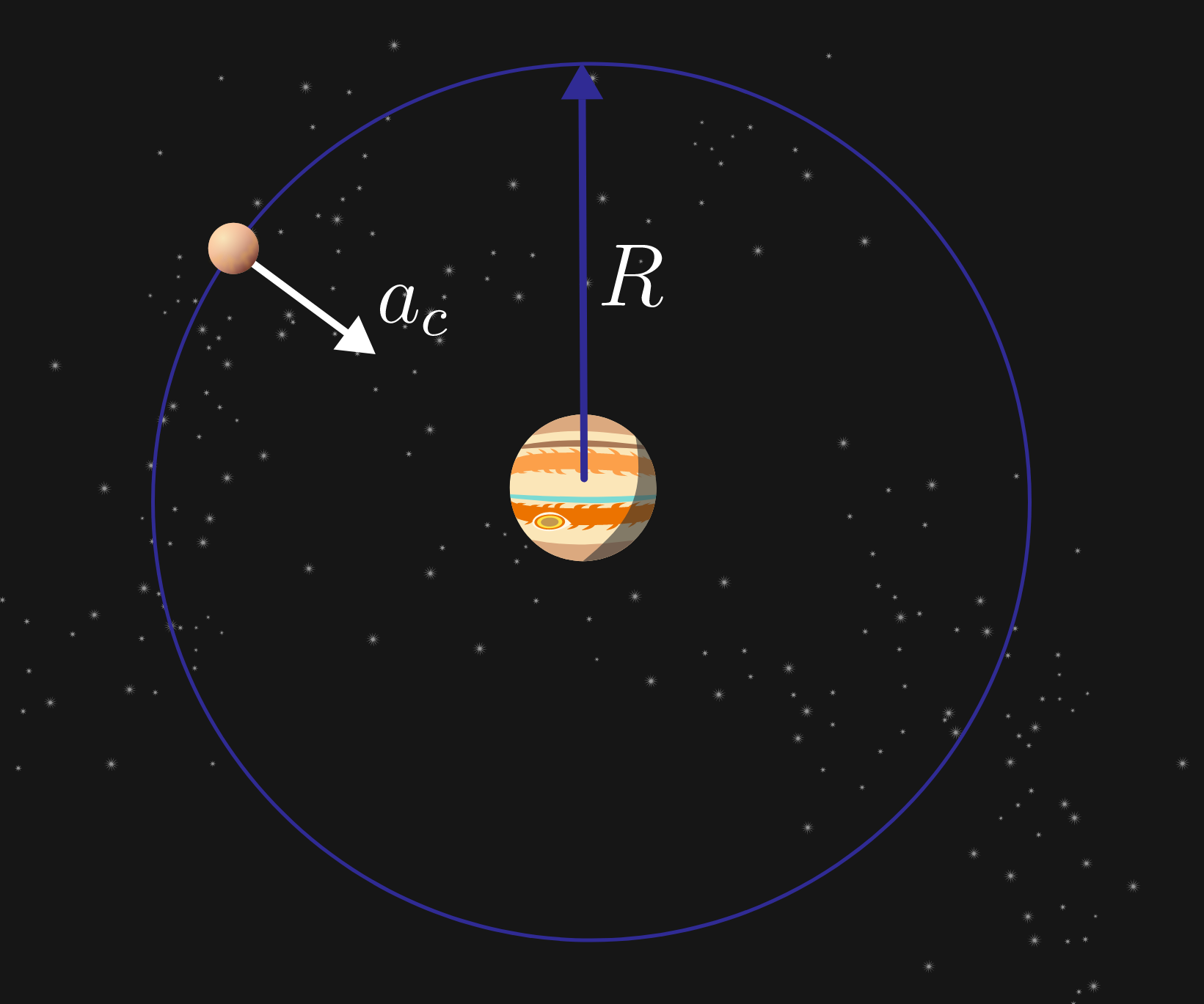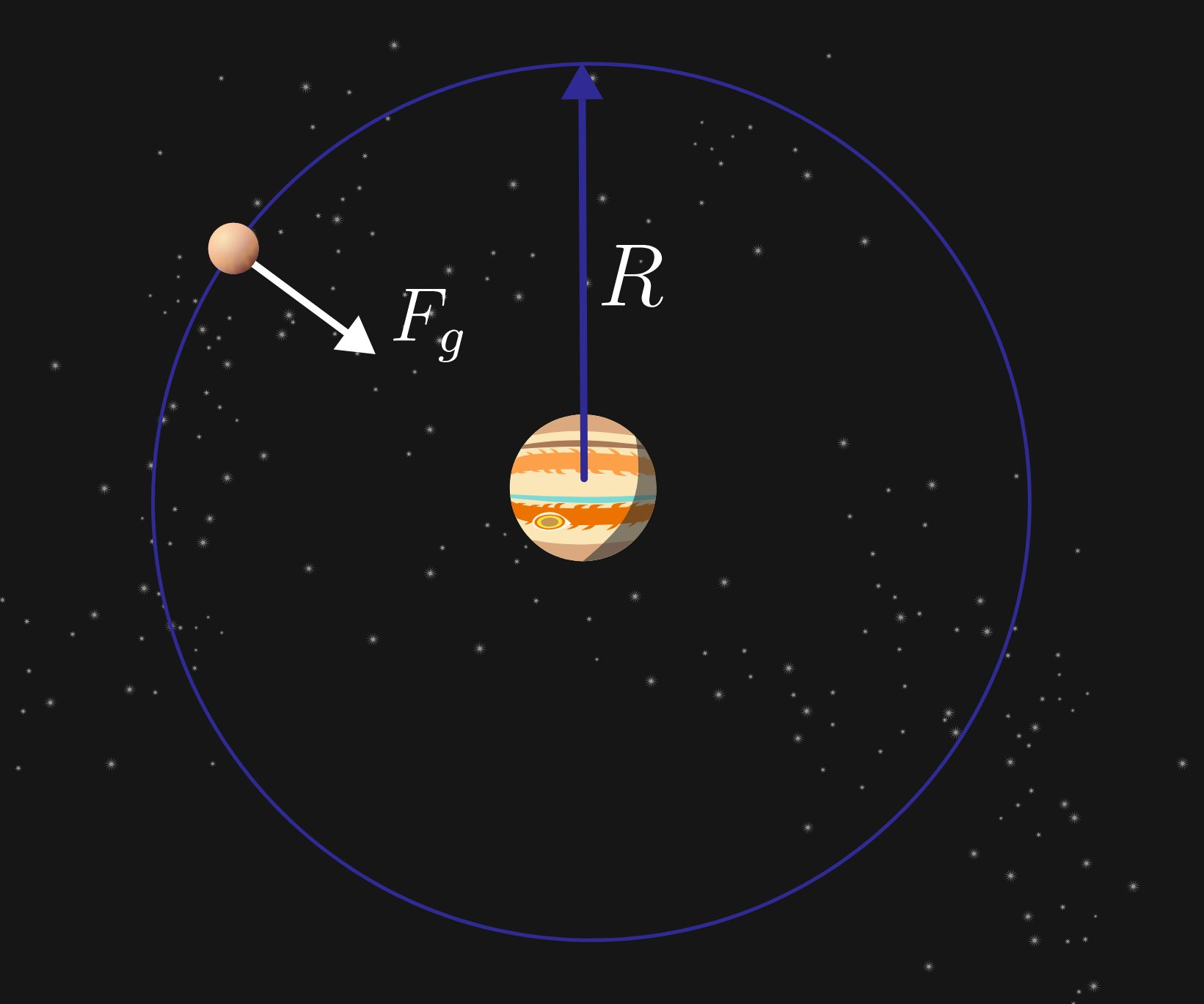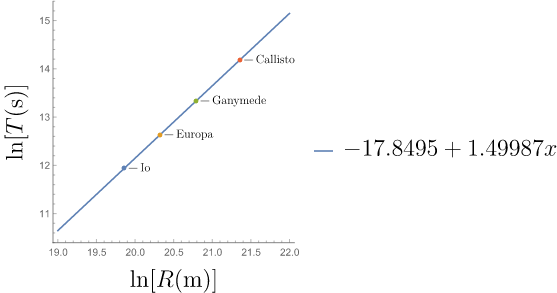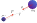### Gravitational Physics

Newton's inverse-square force law

$F_g = \frac{GM_1 M_2}{r^2}$

is elegant in its simplicity and leads to predictions qualitatively similar to observations, but it does not graduate to become a physical theory until ample quantitative data supports it.

In this quiz, we will investigate how scientists resolve theoretical models with experimental data. Specifically, we test the hypothesis that $F_g$ is proportional to $1/r^2$ by examining a set of measurements of the orbital periods and radii of Jupiter's moons.

$\begin{array}{c|c} \text{Moon} & \text{Orbital period}\,T\,(10^6 \si{\second}) & \text{Orbital radius}\,R\,(10^6 \si{\kilo\meter})\\ \hline \text{Io}& 0.153 & 0.422 \\ \text{Europa}& 0.307& 0.671 \\ \text{Ganymede}& 0.618& 1.070\\ \text{Callisto}& 1.442& 1.883 \\ \end{array}$

In the next few questions we will

• first, using Newton's gravitational force law, derive a relationship between orbital period and orbital radius for one of Jupiter's moons
• then, perform a linear regression—the workhorse of $19^\text{th}$ and $20^\text{th}$ century science—which returns model parameters that most closely reproduces one variable as a function of the other.

# Apply: Heavenly Orbits

Possibly the most challenging step in validating a theoretical model with observational data is deciding on which data are most directly relevant. Here, we have basically identified that orbital radius and period are to be used as a test of Newton's gravitational law.

How Newton and concurrent astronomers whittled down all possible data to these two variables is too arduous to describe here. Clearly, directly measuring the force between Jupiter and any of its moons is not feasible. It helped that these data are accessible to any patient astronomer with a decent telescope. Our task is to determine how Newton's gravitational law relates these variables.The orbital period $T$ is how long it takes for one of Jupiter's moons to complete one revolution around the planet, but time does not appear explicitly in $F_g.$ Modeling our approach on the last quiz where we related the Moon's centripetal acceleration to $F_g$ let's look for a way to pull $T$ into this relationship.

If the orbital radius is $R$ which choice expresses centripetal acceleration $a_c = v^2/R$ in terms of $T?$

# Apply: Heavenly Orbits

According to the result of the last quiz, we suspect that $F_g\propto 1/r^2$ but we would like to assess whether data from Jupiter's moons supports the same theory.

Thus, let's introduce a parameter $\epsilon$ into the force law

$F_g=\frac{Gm_\text{moon}M_\text{Jup.}}{r^{2+\epsilon}}$

expressing the deviation of the power law from an inverse-square law. We will use the data to estimate $\epsilon.$ The closer $\epsilon$ is to $0,$ then the stronger the support for Newton's inverse-square law.

Using the result of the previous question, and Newton's second law, which choice expresses orbital period $T$ in terms of orbital radius $R?$Details

• For a moon in a circular orbit, $F_g=m_\text{moon} a_c.$

• Assume the moons' orbits are circular and their motion is uniform.

# Apply: Heavenly Orbits

In a linear regression, the calculated parameters are the slope and intercept of a line that best fits a set of data.

Plotting the natural log $(\ln)$ of period $T$ and orbital radius $R$ of the moon data, they fall almost exactly on a line. The slope and intercept of the best fit line are shown next to the plot.Taking the natural log of your model relationship between $T$ and $R$ in the previous problem, what is the order of magnitude of the deviation $\epsilon?$

# Apply: Heavenly Orbits

Given that Jupiter's mass is $\SI{1.898e27}{\kilo\gram}$, make a prediction for proportionality constant $G$ from this data.The accepted value of the proportionality constant in Newtonian gravity is $G=\SI[per-mode=symbol]{6.67408e-11}{\meter\cubed\per\kilo\gram\per\second\squared}.$ By what fraction does the predicted value deviate from the accepted value?

Calculate $\frac{\lvert G_\text{measured}-G \rvert}{G}.$

# Apply: Heavenly Orbits

Orbital data has been used over the last several centuries to validate Newton's universal law of gravity between massive bodies, as well as to validate its only known improvement (general relativity). Here, we've used it to narrow down the functional form of gravity to within one part in $\num{10000}$ of the exponent on $r.$

We can summarize our findings as follows:

Newtonian gravity is defined by the force law $F = G \frac{m_1 m_2}{r^2},$ where $r$ is the magnitude of the displacement of the centers of two masses $m_1$ and $m_2,$ with $G=\SI{6.67e-11}{\meter\cubed\per\kilo\gram\per\second\squared}.$ The force between the masses is always attractive, directed between the centers of $m_1$ and $m_2.$# Apply: Heavenly Orbits

Newton's work unearthed the connection between falling objects and orbital motion. His theory formed the foundation of orbital mechanics, more than $250$ years before the first man-made object was launched into orbit in $1957.$ Today, with more than $2000$ man-made satellites in orbit around Earth (and even more space junk), Newton's theory remains more relevant today than ever.

Newton's theory withstands the test of time because it exemplifies a bedrock assumption of modern science: that the laws of nature are systematic and universal, which was not a widely held belief in his time.

×